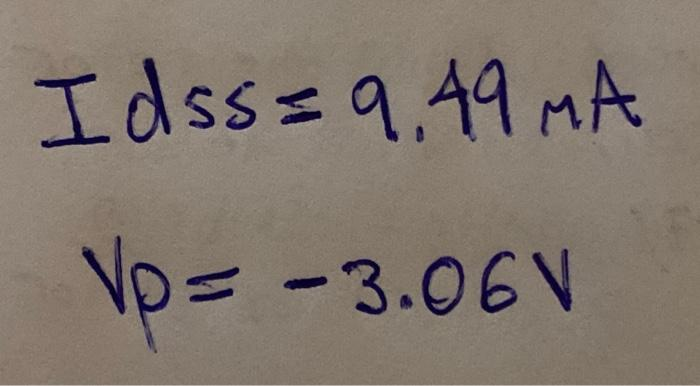# Question b. Using the loss and Vp determined in Part 1, sketch the transfer characteristics in Fig. 14.3. (MA) Figure 14-3 Transfer characteristic for the 2N4416 JFET. c. The choice of ID, - Vidss) will permit a maximum swing in collector current for the AC domain. Draw a horizontal line from Klipss) on the Ic axis to the transfer curve of Fig. 14.3 and label the intersection as the Q-point to define the self-bias line. Label the resulting line as the self-bias line for future reference. d. Calculate the value of Rg from AVOS Rsal ΔΙ where I specifies the magnitude of the quantity and the change in each quantity from the origin to the Q-point. Idss=9,49 MA No= -3.06V VpSET1W4 The Asker · Electrical EngineeringTranscribed Image Text: b. Using the loss and Vp determined in Part 1, sketch the transfer characteristics in Fig. 14.3. (MA) Figure 14-3 Transfer characteristic for the 2N4416 JFET. c. The choice of ID, - Vidss) will permit a maximum swing in collector current for the AC domain. Draw a horizontal line from Klipss) on the Ic axis to the transfer curve of Fig. 14.3 and label the intersection as the Q-point to define the self-bias line. Label the resulting line as the self-bias line for future reference. d. Calculate the value of Rg from AVOS Rsal ΔΙ where I specifies the magnitude of the quantity and the change in each quantity from the origin to the Q-point. Idss=9,49 MA No= -3.06V Vp
More
Transcribed Image Text: b. Using the loss and Vp determined in Part 1, sketch the transfer characteristics in Fig. 14.3. (MA) Figure 14-3 Transfer characteristic for the 2N4416 JFET. c. The choice of ID, - Vidss) will permit a maximum swing in collector current for the AC domain. Draw a horizontal line from Klipss) on the Ic axis to the transfer curve of Fig. 14.3 and label the intersection as the Q-point to define the self-bias line. Label the resulting line as the self-bias line for future reference. d. Calculate the value of Rg from AVOS Rsal ΔΙ where I specifies the magnitude of the quantity and the change in each quantity from the origin to the Q-point. Idss=9,49 MA No= -3.06V Vp